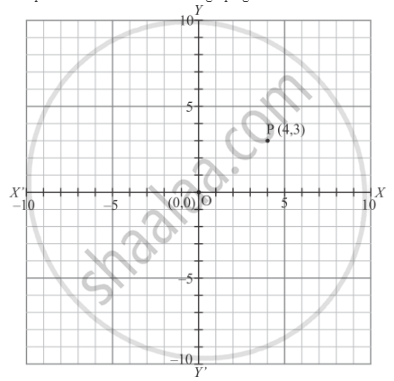# The Perpendicular Distance of the Point P (4, 3) from X-axis is - Mathematics

MCQ

The perpendicular distance of the point P (4, 3) from x-axis is

#### Options

• 4

• 3

• 5

• none of these

#### Solution

The point  P(4,3 ) is shown in the graph given below:Thus the perpendicular distance of the point  P(4,3 ) from x−axis is 3 units.

Concept: Coordinate Geometry
Is there an error in this question or solution?

#### APPEARS IN

RD Sharma Mathematics for Class 9
Chapter 8 Co-ordinate Geometry
Exercise 8.2 | Q 11 | Page 7

Share